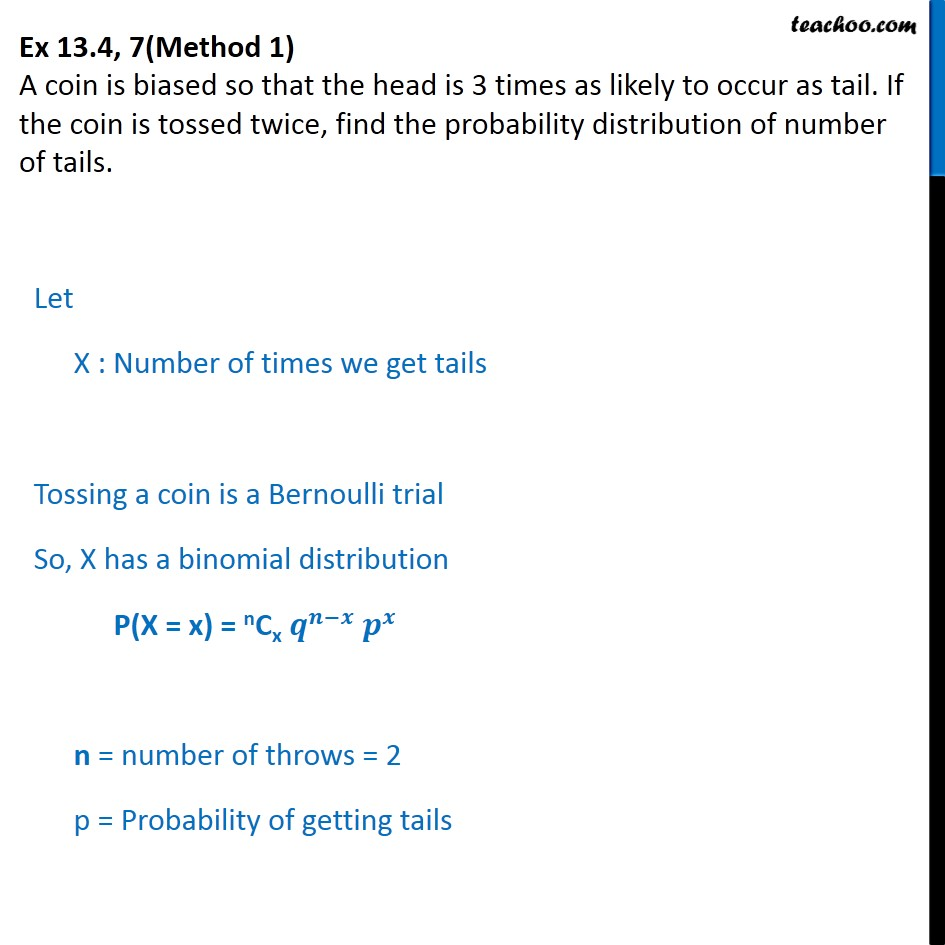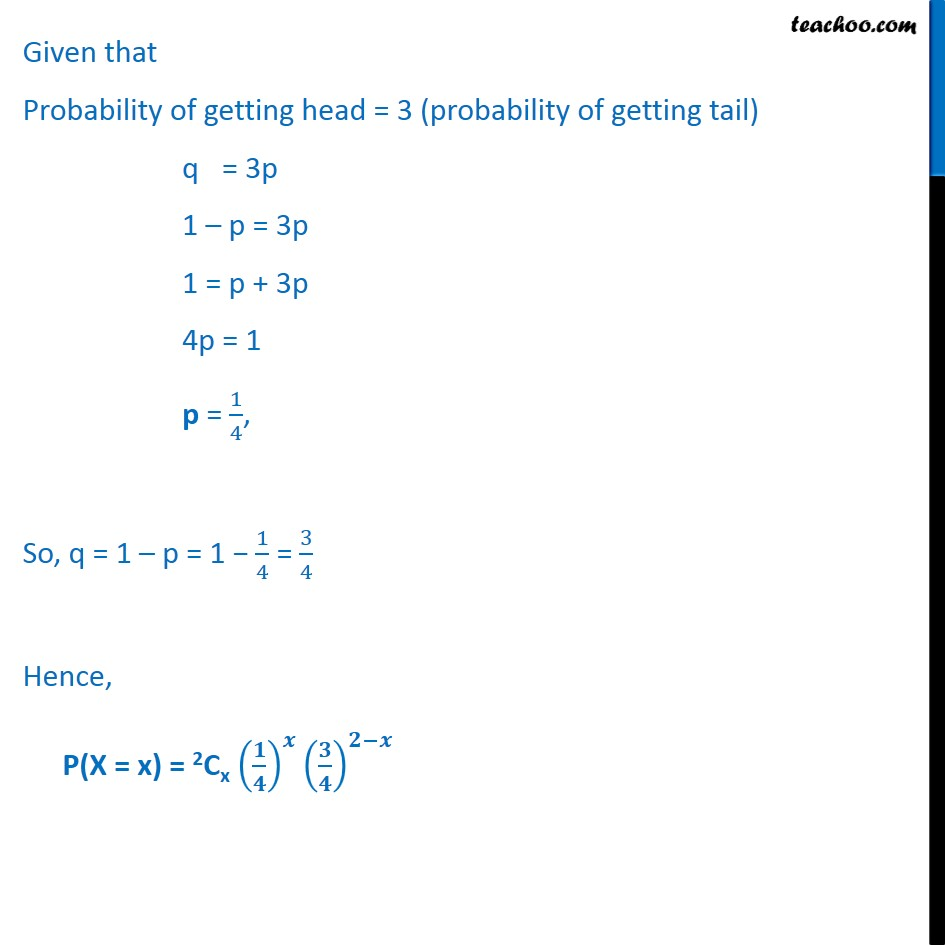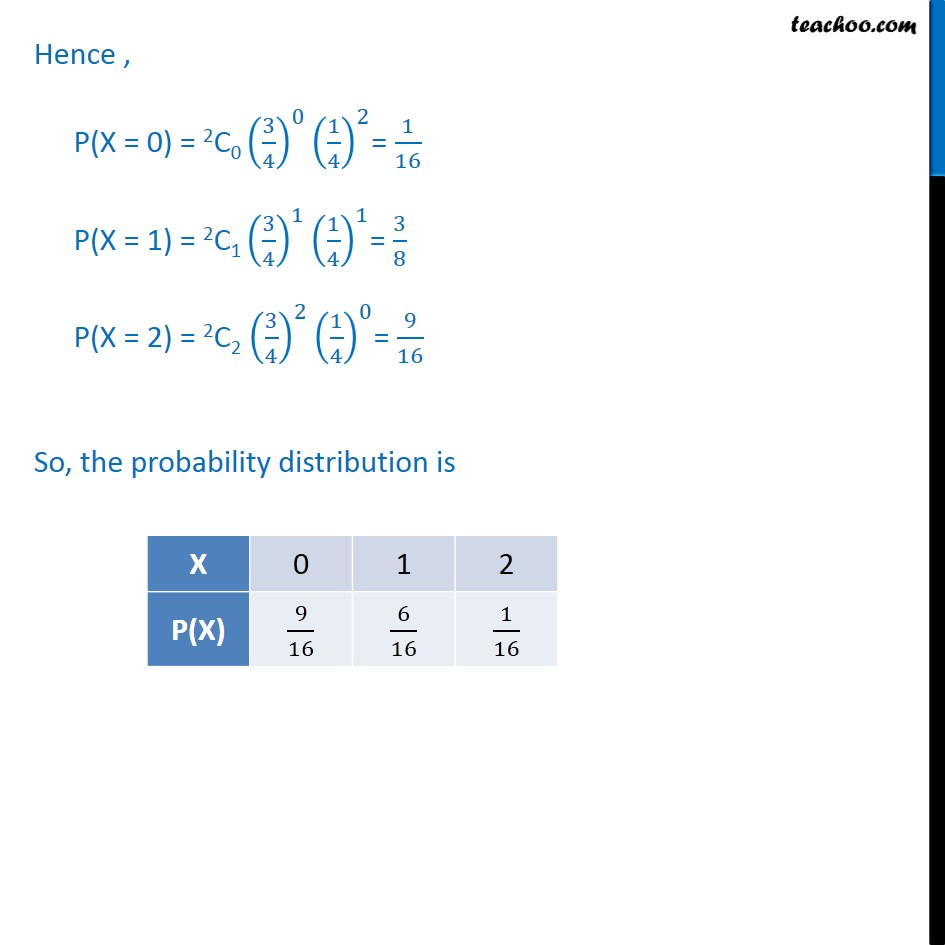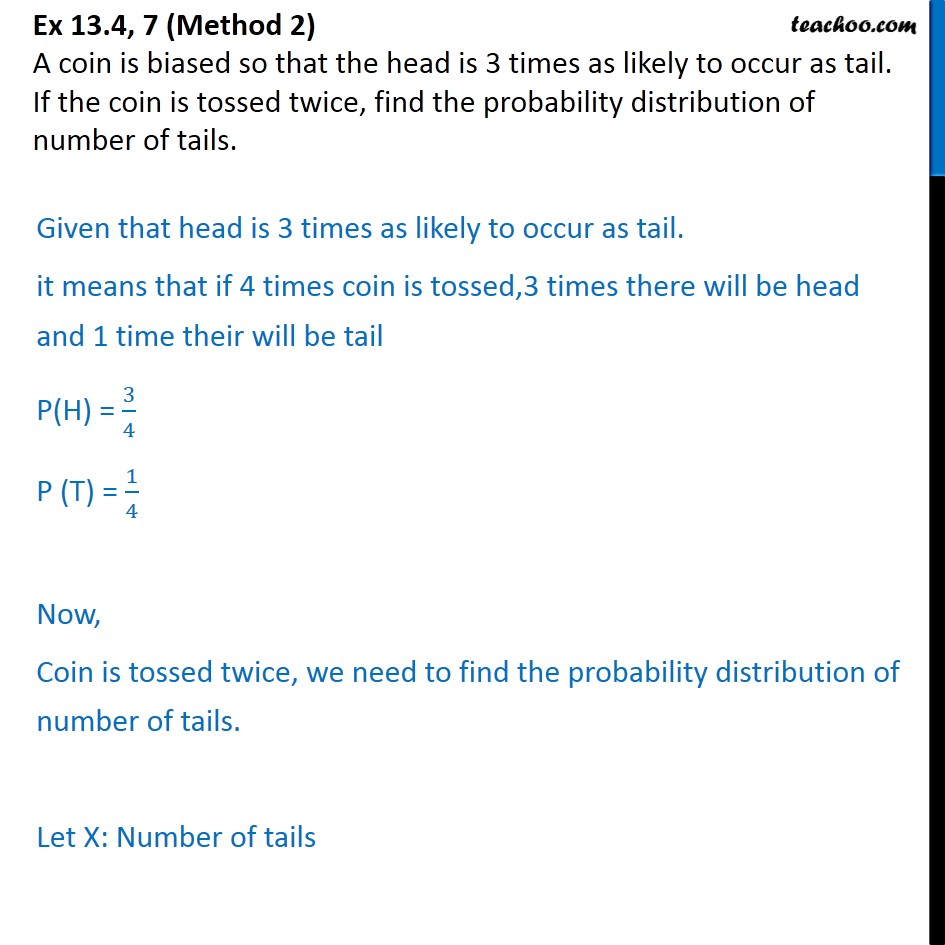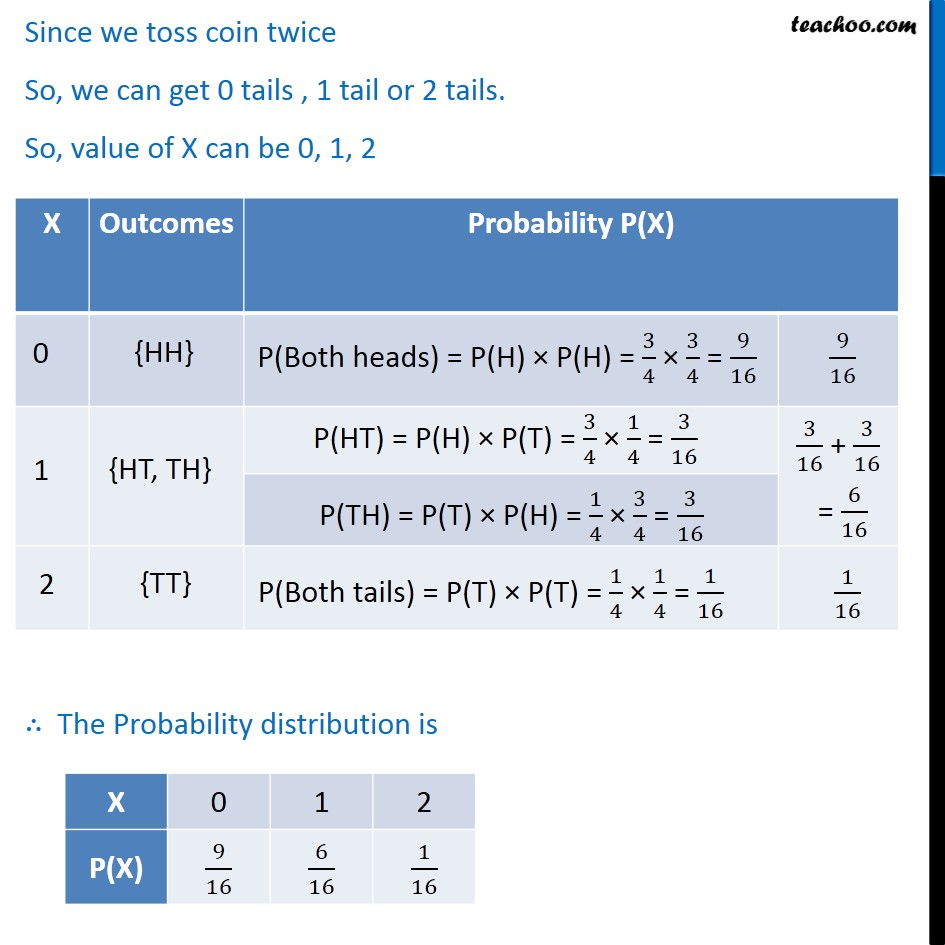1. Chapter 13 Class 12 Probability
2. Serial order wise
3. Ex 13.4

Transcript

Ex 13.4, 7(Method 1) A coin is biased so that the head is 3 times as likely to occur as tail. If the coin is tossed twice, find the probability distribution of number of tails. Let X : Number of times we get tails Tossing a coin is a Bernoulli trial So, X has a binomial distribution P(X = x) = nCx 𝒒﷮𝒏−𝒙﷯ 𝒑﷮𝒙﷯ n = number of throws = 2 p = Probability of getting tails Given that Probability of getting head = 3 (probability of getting tail) q = 3p 1 – p = 3p 1 = p + 3p 4p = 1 p = 1﷮4﷯, So, q = 1 – p = 1 − 1﷮4﷯ = 3﷮4﷯ Hence, P(X = x) = 2Cx 𝟏﷮𝟒﷯﷯﷮𝒙﷯ 𝟑﷮𝟒﷯﷯﷮𝟐−𝒙﷯ Hence , P(X = 0) = 2C0 3﷮4﷯﷯﷮0﷯ 1﷮4﷯﷯﷮2﷯= 1﷮16﷯ P(X = 1) = 2C1 3﷮4﷯﷯﷮1﷯ 1﷮4﷯﷯﷮1﷯= 3﷮8﷯ P(X = 2) = 2C2 3﷮4﷯﷯﷮2﷯ 1﷮4﷯﷯﷮0﷯= 9﷮16﷯ So, the probability distribution is Ex 13.4, 7 (Method 2) A coin is biased so that the head is 3 times as likely to occur as tail. If the coin is tossed twice, find the probability distribution of number of tails. Given that head is 3 times as likely to occur as tail. it means that if 4 times coin is tossed,3 times there will be head and 1 time their will be tail P(H) = 3﷮4﷯ P (T) = 1﷮4﷯ Now, Coin is tossed twice, we need to find the probability distribution of number of tails. Let X: Number of tails Since we toss coin twice So, we can get 0 tails , 1 tail or 2 tails. So, value of X can be 0, 1, 2 ∴ The Probability distribution is

Ex 13.4